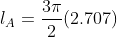Trigonometry : Arc Length

Example Questions

Example Question #1 : Arc Length

Which is true of the relationship between the arc measure and the central angle as shown below?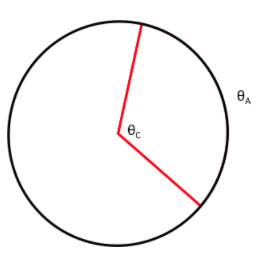The arc length is half of the central angle

The central angle is half of the arc length

The central angle will always be a right angle

They are equal

They are equal

Explanation:

Every arc has a measure that is equal to the measure of the central angle that creates the arc.  This is because the measure of the angle determines the distance around the circumference that the arc makes.

Example Question #11 : Angle Applications

Which of the following is the correct formula for finding arc length?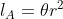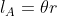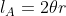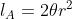Explanation:

The circumference of an entire circle is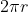.  When considering the length of an arc, the angle is less than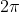denoted by angle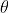. So the formula for finding the length of an arc is replacing the angle of an entire circle,, with the angle that forms the arc,.  This gives us the formula.

Example Question #3 : Arc Length

Which of the following is the correct arc length formed by the angle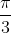of a circle whose radius is a length of 5?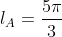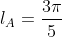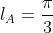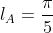Explanation:

We must use the formula for finding arc length.  We have been given all the information needed to just plug into the formula.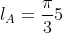Example Question #4 : Arc Length

Which of the following is the correct arc length formed by an angle with measure 30 degrees of a circle whose radius is a length of 3?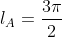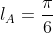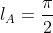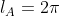Explanation:

First, we are given our angle measure in degrees and we must convert to radians to be able to use our arc length formula.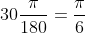Now we are able to plug the radius length and the angle measure into our formula and solve for the arc length.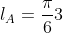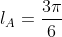Example Question #21 : Angle Applications

What is the measure of the angle that forms an arc with length 2.33 of a circle who has radius 4?  Round to the second decimal place.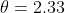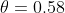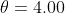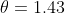Explanation:

We must use the formula for finding arc length to solve for the measure of the angle,.  The formula is.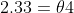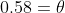Example Question #6 : Arc Length

An arc has a measure of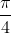and a diameter of 7, what is the measure of the central angle?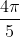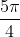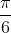Explanation:

Notice the question gave you the measure of the arc, NOT the arc length.  The measure of an arc is equal to the measure of the central angle that forms the arc.  We do not even need to use our formula for this one, just the fact that the central angle is equal to the measure of the arc that it forms.

Example Question #7 : Arc Length

True or False: You are given the central angle measure but no other information, you are able to solve for the arc length.

False

True

False

Explanation:

We do not have enough information to solve for the arc length.  We know that the measure of the arc is equal to the central angle and that the measure of the arc is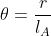but we still have two unknowns with no method to solve for them.

Example Question #8 : Arc Length

You know that the central angle of a sector is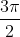and the sector area is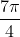, what is the arc length?  Round to two decimals.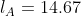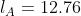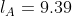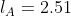Explanation:

The formula to find the area of a sector is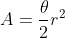.  The piece of information we are missing to solve for arc length is the radius, so we will use the formula for finding the area of a sector to solve for radius, allowing us to solve for the arc length.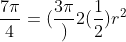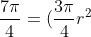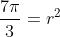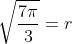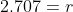Now we can plug this into our formula to solve for arc length